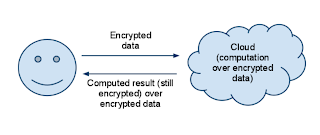## Wednesday, March 17, 2010

### Computation over encrypted data [Crypto]

The following diagram shows the ideal situation:The objective is to perform a general computation over encrypted data so that the party that performs the computation learns neither the input values nor the result of the computation (over a finite field). The computation to be performed (e.g. eigenvalue computation, null space computation, Gaussian elimination, etc.) is public (i.e. known to everyone). Theoretically speaking, one can achieve the above objective using a SMC (Secure Multiparty Computation) protocols by evaluating a scrambled Boolean circuit. However, it is not practical.

Two popular practical techniques that we can use:
1. Commutative encryption (Pohlig-Hellman)
2. Homomorphic encryption (Paillier, Damgard, Unpadded RSA, Benaloh, ElGamal, etc.)

Since I am interested in one off computation, IMO, homomorphic encryption is the most suitable here. Computations over finite fields, in general, involves two binary operations (e.g. addition and multiplication). However, all the practical homomorphic crypto systems are homomorphic to only one operation. (E.g.: addition - Paillier, Damgard, Benaloh; multiplication - Unpadded RSA, Elgamal). It should be noted that mid last year, IBM published a paper on a fully homomorphic encryption using ideal lattices, but it is computationally intensive and thus not suitable for real applications. So, it is still an open problem to invent a practical fully homomorphic encryption. Until such an invention, we need to rely on specialized protocols to solve the afore mentioned problem.

#### 1 comment:

Natalia said...

Thanks for explaining these two approaches that are used to perform computation over encrypted data. Since I am learning about these technique the very first time so I am not very much cleared with the logic.
electronic signature